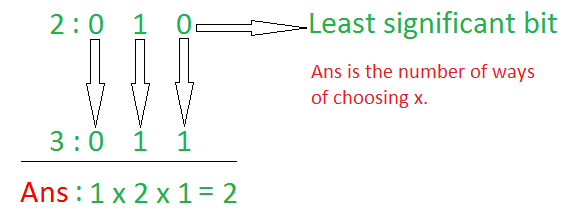Related Articles

# Count values whose Bitwise OR with A is equal to B

• Last Updated : 14 Jun, 2021

Given two integers A and B, the task is to count possible values of X that satisfies the condition A | X = B.
Note: | represents Bitwise OR operation.

Examples:

Input: A = 2, B = 3
Output: 2
Explanation:
Since, 2 | 1 = 3 and 2 | 3 = 3. Therefore, the possible values of x are 1 and 3.

Input: A = 5, B = 7
Output: 4

Naive Approach: The simplest approach to solve this problem is to iterate over the range [1, B] and check for every number, whether its Bitwise OR with A is equal to B. If the condition is satisfied, increment the count.
Time Complexity: O(b)
Auxiliary Space: O(1)

Efficient Approach: The above approach can be optimized based on the following observations:

• Convert the numbers A and B into their respective binary representations.

Truth table of Bitwise OR operation:
1 | 1 = 1
1 | 0 = 1
0 | 1 = 1
0 | 0 = 0

• For each same-positioned bit, count number of ways the ith bit in A can be converted to the ith bit in B by performing Bitwise OR operation.Follow the steps below to solve the problem:

• Initialize a variable ans to store the required result.
• Traverse the bits of a and b simultaneously using the variable i
• If the ith bit of a is set and the ith bit of b is also set, then the ith bit of x can take 2 values, i.e, 0 or 1. Hence, multiply the ans by 2.
• If the ith bit of a is unset and the ith bit of b is set, then the ith bit of x can take only 1 value, i.e, 1. Hence, multiply the ans by 1.
• If the ith bit of a is set and the ith bit of b is unset, then no matter what the ith bit of x is, the ith bit of a can not be converted to ith bit of b. Hence, update ans to 0 and break out of the loop.
• Print the value of ans as the result

Below is the implementation of the above approach:

## C++

 `// C++ program for the above approach``#include ``using` `namespace` `std;`` ` `// Function to count possible values of``// X whose Bitwise OR with A is equal to B``int` `numWays(``int` `a, ``int` `b)``{`` ` `    ``// Store the required result``    ``int` `res = 1;`` ` `    ``// Iterate over all bits``    ``while` `(a && b) {`` ` `        ``// If i-th bit of a is set``        ``if` `((a & 1) == 1) {`` ` `            ``// If i-th bit of b is set``            ``if` `((b & 1) == 1) {`` ` `                ``// The i-th bit in x``                ``// can be both 1 and 0``                ``res = res * 2;``            ``}``            ``else` `{`` ` `                ``// a|x is equal to 1``                ``// Therefore, it cannot``                ``// be converted to b``                ``return` `0;``            ``}``        ``}`` ` `        ``// Right shift a and b by 1``        ``a = a >> 1;``        ``b = b >> 1;``    ``}`` ` `    ``return` `res;``}`` ` `// Driver Code``int` `main()``{``    ``// Given Input``    ``int` `a = 2, b = 3;`` ` `    ``// Function Call``    ``cout << numWays(a, b);`` ` `    ``return` `0;``}`
Output:
```2
```

Time Complexity: O(max(log a, log b))
Auxiliary Space: O(1)

Attention reader! Don’t stop learning now. Get hold of all the important DSA concepts with the DSA Self Paced Course at a student-friendly price and become industry ready.  To complete your preparation from learning a language to DS Algo and many more,  please refer Complete Interview Preparation Course.

In case you wish to attend live classes with experts, please refer DSA Live Classes for Working Professionals and Competitive Programming Live for Students.

My Personal Notes arrow_drop_up